# Hearing the Clusters of a Graph¶

We replicate the results of https://arxiv.org/abs/0911.4729 - "Hearing the clusters in a graph: A distributed algorithm" for clustering a simple line graph.

With our implementation, we are unable to cluster datasets like a mixture of 8 well separated Gaussians.

In :
import numpy as np
from lib.spectral_clustering import similarity_matrix, laplacian_matrix, spectral_clustering
from lib.datasets import gaussian_mixture as gm
from lib.kmeans import kmeans
import matplotlib.pyplot as plt
import numpy as np
import scipy as sp
from matplotlib import cm
import scipy.fftpack
from lib.wave_clustering import wave_clustering
import scipy.signal
import gif


# Building Our Functions to Cluster with the Wave Equation¶

In :
def array_to_binary(row):
'''
This function takes a row of 0's and 1's and turns it into binary,
backwards, so [0, 0, 1] becomes 4
'''
binary_to_decimal = 0
for i in range(len(row)):
binary_to_decimal += row[i] * 2 ** i
return binary_to_decimal

def cluster_wave_eq(k, T_max, num_nodes, c, normalized_laplacian):
'''
k = number of eigenvectors used, maybe??? < 2**k clusters
T_max = number of iterations in time
num_nodes = number of nodes in our graph
c = constant in the wave equation. 0 < c < sqrt(2) for stability
normalized_laplacian = D**{-1}L
'''
# initialize heat matrix: each row corresponds to a node, column corresponds to a step in time
heat = np.zeros(shape = (num_nodes, T_max + 1)) #offset index by 1 because we need index -1, evolves with time
#print(heat.shape)
heat[:, 0] = np.random.uniform(size = num_nodes)
heat[:, 1] = heat[:, 0]
#print(heat[:, 1].shape)
# populate the heat matrix
for i in range(2, T_max + 1):
heat[:, i] = 2 * heat[:, i-1] - heat[:, i-2] - (c ** 2) * (normalized_laplacian @ heat[:, i-1])
# calculate FFT for each node, the nth entry corresponds to e^(2pi ni/N) and encodes amplitude and phase shift
heat_fft = np.fft.fft(heat, axis=1)
# Finds the index of the k largest power over each row
indices = [sp.signal.find_peaks(heat_fft[j, :])[:k] for j in range(len(heat_fft))]
eigenvector = np.zeros(shape = (num_nodes, k))
binary = np.zeros(shape = (num_nodes, k))
for i in range(num_nodes): # for each row, ie each node
for j in range(k-1): # find the jth largest frequency
eigenvector[i][j] = np.real(heat_fft[i][j])
if eigenvector[i][j] > 0:
binary[i][j] = 1
else:
binary[i][j] = 0
cluster_num = [array_to_binary(binary[i]) for i in range(num_nodes)]
return cluster_num


# Generating Data¶

In :
n_gaussians = 8
n_pts = 32
n = n_pts * n_gaussians
d = 2

data = gm(n_gaussians, n_pts, d, centroid_var=10)

plt.scatter(*data.T)
plt.xlabel("$x_1$")
plt.ylabel("$x_2$")
plt.title("Sample of Gaussian Mixture")
plt.show()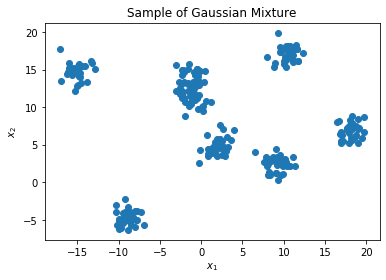# Standard Graph Spectral Clustering¶

In :
spectral = spectral_clustering(data, k=n_gaussians, lform="rw", metric = "g", s=1)
for i in range(len(spectral)):
d = data[spectral == i].T
x = d
y = d
plt.scatter(x,y)
plt.title("Standard Spectral Clustering")
plt.show()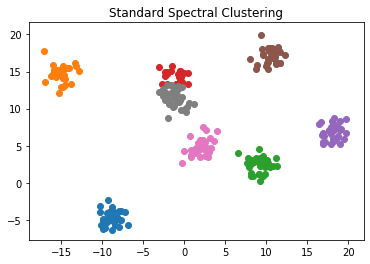# Wave Equation Clustering¶

In :
spectral = wave_clustering(data, k=8, T_max=10000, c=0.1, metric = "g", dynamics_gif_path="Dyanmics.gif")
for i in range(len(spectral)):
d = data[spectral == i].T
x = d
y = d
plt.scatter(x,y)
plt.title("Wave Equation Clustering")
plt.show()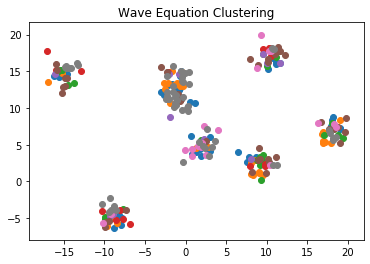# Line Graph Clustering¶

In :
S = np.diag(np.ones(199), k=1) + np.diag(np.ones(199), k=-1)
S[100, 101] = 0.1
S[101, 100] = 0.1
L, deg = laplacian_matrix(S)
L_norm = (1/deg).reshape((-1, 1)) * L

k = 1
T_max = 1000
c = 1.41

In :
n = len(L)

def dctmtx(n, normalize=False):
d = sp.fftpack.dct(np.eye(n), axis=0)
if(normalize):
d = d / np.sqrt(np.sum(d**2, axis=1))
return d

def find_extrema(vec):
# a peak is a local min or local max
# ie either greater than both neighbors, or less than both neighbors
forward_diffs = vec[:-1] - vec[1:]
backward_diffs = vec[1:] - vec[:-1]
above_f_neighbor = np.concatenate(( (forward_diffs > 0), [False]) )
above_b_neighbor = np.concatenate(( [False], backward_diffs > 0 ) )
return np.where(np.logical_not(np.logical_xor(above_f_neighbor, above_b_neighbor)))


Use fft and rfft on cosine waves to debug cos coefficient computations. Make sure peaks of the M matrix matches up, peaks should match. Be sure that fft identifies pure frequency components, eg exactly periodic. Post process fourier coefficients and combine frequency bases to get cos sin basis.

# Graph Laplacian Eigenfunctions for Line Graph¶

In :
n = 500

line_graph_sim = np.diag(np.ones(n-1), k=1) + np.diag(np.ones(n-1), k=-1)
laplacian, diag = laplacian_matrix(line_graph_sim)
l_rw = (1/diag)[:, None] * laplacian

In :
evals, evecs = sp.linalg.eigh(laplacian, np.diag(diag))

In :
plt.plot(evals)
plt.title("Eigenvalues")
plt.xlabel("Index")
plt.ylabel("Value")
plt.show()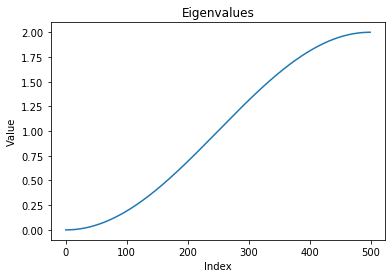In :
plt.plot(evecs[:, :2])
plt.title("Eigenvectors")
plt.xlabel("Coordinate")
plt.ylabel("Value")
plt.show()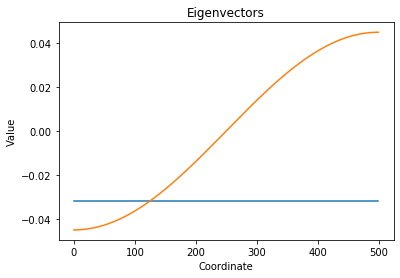Goal: do the eigenfunctions pop out of a time series on the graph?

When we solve the heat equation using separation of variables, we are actually isolating dominant frequency components of a diffused signal. These dominant components don't involve time because of the separation of variables setup.

In :
@gif.frame
def plot(i):
plt.plot(evecs[:, :i])
plt.title("Eigenvectors")
plt.xlabel("Coordinate")
plt.ylabel("Value")
frames = []
for i in range(2, 10):
frames.append(plot(i))
gif.save(frames, 'example.gif', duration=50)

In :
def wave_clustering(data_sim, k, T_max=1000, c=1.41, metric=None, kernel=None, dynamics_gif_path=None, **kwargs):
'''
Args:
data (np.ndarray): (n,d) numpy array consisting of n d-valued points
k (integer): desired number of clusters. Must be a power of 2.
T_max: number of iterations of wave propagations to simulate. A larger value is slower but provides more accurate
estimation of laplacian eigenvectors.
c: wave propagation speed. 0 < c < sqrt(2) is required for guaranteed stability.
metric: one of ["g", "e", "k"] - choose a metric for the data:
"g" for Gaussian: d(x, y) = exp(-|x-y|^2 / 2 (s^2)). The scale s controls standard deviation.
"e" for Exponential: d(x, y) = exp(-|x-y|/s). The scale s is the parameter of the exponential.
"eps" for Epsilon neighbors: d(x, y) = |x-y| if less than epsilon, 0 otherwise
"k" for Kernel: use an arbitrary kernel, given by the kernel argument.
kernel: K(x, y, s) -> \R+ - an arbitrary distance function between two points of the same dimension with a given scale.
dynamics_gif_path (str): if provided, save a gif at the given path showing the time evolution of the system
Returns:
A list of (n,) integers of the cluster assignments of each data point.
'''
k_ = int(np.log2(k))
assert k_ == np.log2(k), "k must be a power of 2"

#data_sim = similarity_matrix(data, metric=metric, kernel=kernel, **kwargs)
n, _ = data_sim.shape
laplacian, degree = laplacian_matrix(data_sim)
rw_laplacian = (1/degree).reshape((-1, 1)) * laplacian

wave = np.zeros((n, T_max + 1))

wave[:, 0] = np.random.uniform(size=n)
wave[:, 1] = wave[:, 0]

frames = []

@gif.frame
def plot(i):
plt.plot(wave[:, i])
plt.xlabel("Graph Node")
plt.ylabel("Function Value")
plt.ylim((np.min(wave), np.max(wave)))

for i in range(2, T_max + 1):
wave[:, i] = 2 * wave[:, i-1] - wave[:, i-2] - (c**2) * (rw_laplacian @ wave[:, i-1])

if(dynamics_gif_path is not None):
for i in range(2, T_max + 1, 50):
frames.append(plot(i))
gif.save(frames, dynamics_gif_path, duration=50)

wave_fft = np.fft.rfft(wave[:, 1:], axis=1)
wave_cos_coeffs = cos_coeffs(wave_fft)

freq_peaks = [scipy.signal.find_peaks(np.abs(wave_cos_coeffs[idx, 1:])) for idx in range(n)]
truncated_freq_peaks = np.stack([f[:k_] for f in freq_peaks])

cluster_assns = np.zeros((n,))
for i in range(n):
for j, freq_peak in enumerate(truncated_freq_peaks[i]):
if wave_cos_coeffs[i][freq_peak] > 0:
cluster_assns[i] += 2**j
return cluster_assns

def gimme_cos(n, k):
'''
Return a length n column vector whose components are cosine waves of frequency k/(n-1)
'''
return np.cos(2 * np.pi * k * np.arange(n) / n)

def gimme_sin(n, k):
'''
Return a length n column vector whose components are cosine waves of frequency k/(n-1)
'''
return np.sin(2 * np.pi * k * np.arange(n) / n)

def cos_coeffs(fourier_coeffs):
'''
Given a sequence of two-sided fourier coefficients (negative freqs, positive freqs) return the corresponding two-sided cos coeffs
'''
return np.real(fourier_coeffs)

def sin_coeffs(fourier_coeffs):
'''
Given a sequence of two-sided fourier coefficients (negative freqs, positive freqs) return the corresponding two-sided sin coeffs
'''
return -np.imag(fourier_coeffs)